## DFA to Regular Expression-

The two popular methods for converting a given DFA to its regular expression are-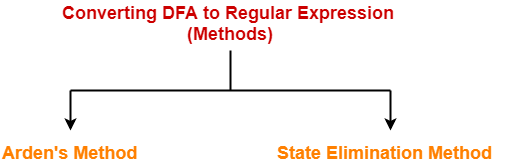1. Arden’s Method
2. State Elimination Method

## Arden’s Theorem-

Arden’s Theorem is popularly used to convert a given DFA to its regular expression.

It states that-

Let P and Q be two regular expressions over ∑.

If P does not contain a null string ∈, then-

R = Q + RP has a unique solution i.e. R = QP*

## Conditions-

To use Arden’s Theorem, following conditions must be satisfied-

• The transition diagram must not have any ∈ transitions.
• There must be only a single initial state.

## Steps-

To convert a given DFA to its regular expression using Arden’s Theorem, following steps are followed-

### Step-01:

• Form a equation for each state considering the transitions which comes towards that state.
• Add ‘∈’ in the equation of initial state.

### Step-02:

Bring final state in the form R = Q + RP to get the required regular expression.

## Important Notes-

### Note-01:

Arden’s Theorem can be used to find a regular expression for both DFA and NFA.

### Note-02:

If there exists multiple final states, then-

• Write a regular expression for each final state separately.
• Add all the regular expressions to get the final regular expression.

## Problem-01:

Find regular expression for the following DFA using Arden’s Theorem-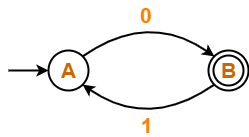## Solution-

### Step-01:

Form a equation for each state-

• A = ∈ + B.1     ……(1)
• B = A.0           ……(2)

### Step-02:

Bring final state in the form R = Q + RP.

Using (1) in (2), we get-

B = (∈ + B.1).0

B = ∈.0 + B.1.0

B = 0 + B.(1.0)          ……(3)

Using Arden’s Theorem in (3), we get-

B = 0.(1.0)*

Thus, Regular Expression for the given DFA = 0(10)*

## Problem-02:

Find regular expression for the following DFA using Arden’s Theorem-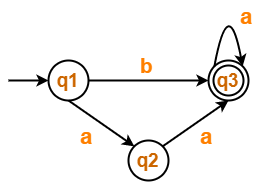## Solution-

### Step-01:

Form a equation for each state-

• q1 = ∈                                        ……(1)
• q2 = q1.a                                   ……(2)
• q3 = q1.b + q2.a + q3.a            …….(3)

### Step-02:

Bring final state in the form R = Q + RP.

Using (1) in (2), we get-

q2 = ∈.a

q2 = a              …….(4)

Using (1) and (4) in (3), we get-

q3 = q1.b + q2.a + q3.a

q3 = ∈.b + a.a + q3.a

q3 = (b + a.a) + q3.a        …….(5)

Using Arden’s Theorem in (5), we get-

q3 = (b + a.a)a*

Thus, Regular Expression for the given DFA = (b + aa)a*

## Problem-03:

Find regular expression for the following DFA using Arden’s Theorem-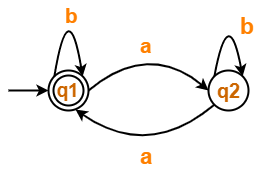## Solution-

### Step-01:

Form a equation for each state-

• q1 = ∈ + q1.b + q2.a                 ……(1)
• q2 = q1.a + q2.b                       ……(2)

### Step-02:

Bring final state in the form R = Q + RP.

Using Arden’s Theorem in (2), we get-

q2 = q1.a.b*             …….(3)

Using (3) in (1), we get-

q1 = ∈ + q1.b + q1.a.b*.a

q1 = ∈ + q1.(b + a.b*.a)          …….(4)

Using Arden’s Theorem in (4), we get-

q1 = ∈.(b + a.b*.a)*

q1 = (b + a.b*.a)*

Thus, Regular Expression for the given DFA = (b + a.b*.a)*

## Problem-04:

Find regular expression for the following DFA using Arden’s Theorem-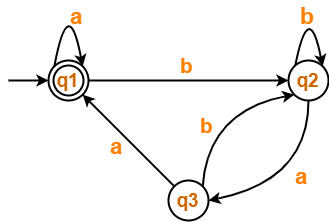## Solution-

### Step-01:

Form a equation for each state-

• q1 = ∈ + q1.a + q3.a                 ……(1)
• q2 = q1.b + q2.b + q3.b            ……(2)
• q3 = q2.a                                  …….(3)

### Step-02:

Bring final state in the form R = Q + RP.

Using (3) in (2), we get-

q2 = q1.b + q2.b + q2.a.b

q2 = q1.b + q2.(b + a.b)          …….(4)

Using Arden’s Theorem in (4), we get-

q2 = q1.b.(b + a.b)*    …….(5)

Using (5) in (3), we get-

q3 = q1.b.(b + a.b)*.a       …….(6)

Using (6) in (1), we get-

q1 = ∈ + q1.a + q1.b.(b + a.b)*.a.a

q1 = ∈ + q1.(a + b.(b + a.b)*.a.a)        …….(7)

Using Arden’s Theorem in (7), we get-

q1 = ∈.(a + b.(b + a.b)*.a.a)*

q1 = (a + b.(b + a.b)*.a.a)*

Thus, Regular Expression for the given DFA = (a + b(b + ab)*aa)*

To gain better understanding about Arden’s Theorem,

Watch this Video Lecture

Next Article- Non-Deterministic Finite Automata

Get more notes and other study material of Theory of Automata and Computation.

Watch video lectures by visiting our YouTube channel LearnVidFun.